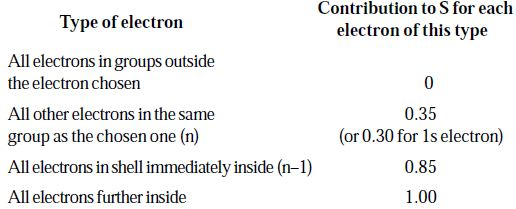# Calculation of Ionic Radii in Slater Rules

The value of screening constant (S) and effective nuclear charge (Z*) can be calculated by using Slater rules. According to these rules the value of “S” for a given electron is estimated as follows.

(i) Write down the complete electronic configuration of the element and divide the electrons into the following orbital groups starting from the inside of the atom.

(1s) : (2s, 2p) : (3s, 3p) : (3d) : (4s, 4p) :

(4d) : (4f) : (5s, 5p) : (6s, 6p) …….etc.

(ii) Select the electron for which the value of S is to be calculated. For this calculation add up the contributions to S for the other electrons according to the following rules.